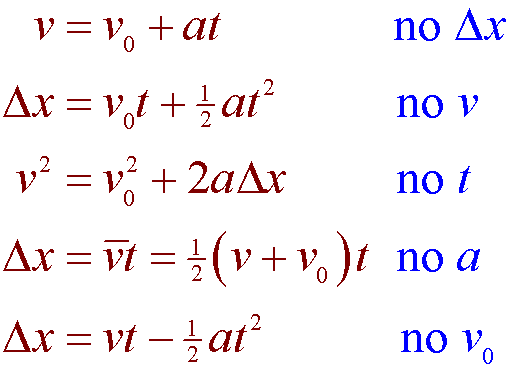User Name Remember Me? Password

 Elementary Math Fractions, Percentages, Word Problems, Equations, Inequations, Factorization, Expansion

 November 9th, 2016, 08:26 PM #1 Newbie   Joined: Aug 2015 From: Alberta Posts: 18 Thanks: 0 Linear Velocity & Acceleration Question I'm having trouble with this question. A car traveling at a speed of 140km/h decelerates for 20 seconds. During this time it travels 500m. a) What is its deceleration? b) What is its speed after the deceleration? Answers in the book are a) 1.389 m/s^2, b) 40 km/h But I can't figure it out.November 9th, 2016, 09:02 PM #2 Math Team   Joined: Jul 2011 From: Texas Posts: 3,016 Thanks: 1600 $\Delta x = v_0 \cdot t + \dfrac{1}{2}at^2$ You were given ... $\Delta x = 500 \, m$, $t=20 \, s$, and $v_0=140 \, km/hr$ You need to convert km/hr to m/s and solve for acceleration, which will be a negative value. Once you determine acceleration, you also have time and initial velocity. use the equation ... $v_f=v_0+at$ Final velocity will be in m/s ... you have to convert it to km/hr.November 23rd, 2016, 01:54 AM   #3
Newbie

Joined: Aug 2015
From: Alberta

Posts: 18
Thanks: 0

Quote:
 Originally Posted by skeeter$\Delta x = v_0 \cdot t + \dfrac{1}{2}at^2$ You were given ... $\Delta x = 500 \, m$, $t=20 \, s$, and $v_0=140 \, km/hr$ You need to convert km/hr to m/s and solve for acceleration, which will be a negative value. Once you determine acceleration, you also have time and initial velocity. use the equation ... $v_f=v_0+at$ Final velocity will be in m/s ... you have to convert it to km/hr.
My m/s I got 140 x 1000 / 3600 to get m/s = 38.89
38.89 / 20 seconds = 1.94 m/s as my Deceleration.... which is off my a bit.

Second questions what do you mean by at?

Displacement / Time = Velocity so. 500 / 20 = 25 m/s Then I divide by 1000 to convert to km's as well as x by 3600

500 / 20 = 25
25 / 1000 = .025
.025 x 3600 = 90 km's

So I'm off there as well

Last edited by jayinwww; November 23rd, 2016 at 01:59 AM.November 23rd, 2016, 04:05 AM #4 Math Team   Joined: Jul 2011 From: Texas Posts: 3,016 Thanks: 1600 You didn't use the equations I cited in my previous post ... fyi, $a \ne \dfrac{v_0}{t}$, it is $a=\dfrac{v_f-v_0}{t}$. You can't use this equation to calculate acceleration because you do not have final velocity. $140 \, km/hr \cdot \dfrac{1000 \, m}{km} \cdot \dfrac{1 \, hr}{3600 \, sec} = \dfrac{350}{9} \, m/s$ $\Delta x = v_0 \cdot t + \dfrac{1}{2}at^2 \implies a= \dfrac{2(\Delta x - v_0 \cdot t)}{t^2}$ $a= \dfrac{2 \left(500 - \frac{350}{9} \cdot 20 \right) }{20^2} = -\dfrac{25}{18} \, m/s^2$ Note $-\dfrac{25}{18} \approx -1.389$ displacement/time is average velocity, not final velocity ... $v_f= v_0 + at$ ... this equation is a direct result of solving the equation $a=\dfrac{v_f-v_0}{t}$ for $v_f$ $v_f = \dfrac{350}{9} + \left(-\dfrac{25}{18}\right) \cdot 20 = \dfrac{100}{9} \, m/s$ $\dfrac{100}{9} \, m/s \cdot \dfrac{3600 \, sec}{1 \, hr} \cdot \dfrac{1 \, km}{1000 \, m} = 40 \, km/hr$ Final note ... if you are studying kinematics under uniform acceleration, you should have been exposed to these equations and the hints on when to use them.Thanks from greg1313 and jayinwww Last edited by skeeter; November 23rd, 2016 at 04:12 AM.Tags acceleration, linear, question, velocityThread ToolsShow Printable VersionEmail this Page Display ModesLinear ModeSwitch to Hybrid ModeSwitch to Threaded ModeSimilar Threads Thread Thread Starter Forum Replies Last Post tankertert Calculus 2 May 9th, 2012 12:40 PM TheSame Calculus 9 May 25th, 2011 06:06 PM JosephE Algebra 2 February 13th, 2010 08:38 AM imcutenfresa Calculus 2 September 15th, 2009 04:11 PM hemi Calculus 3 June 8th, 2009 10:41 AM

 Contact - Home - Forums - Cryptocurrency Forum - Top